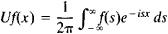# Unitary Operator

(redirected from Unitary operators)
The following article is from The Great Soviet Encyclopedia (1979). It might be outdated or ideologically biased.

## Unitary Operator

an extension of the notation of a rotation of Euclidean space to the infinite-dimensional case. More precisely, a unitary operator is a rotation of a Hilbert space about the origin. In analytic terms, an operator U that maps the Hilbert space H onto itself is said to be unitary if (f, g) = (Uf,Ug) for any two vectors f, g in H. A unitary operator preserves lengths of vectors and angles between vectors and is a linear operator. A unitary operator U has a unitary inverse U–1 such that U–1 = U*, where U* is the adjoint of U.

An example of a unitary operator is the Fourier-Plancherel operator, which associates to each function f(x), – ∞ < x < ∞, with square integrable absolute value the functionReferences in periodicals archive ?
In the quantum context, Uhlmann proved that if [rho], [sigma] [member of] S(H) and dim H < [infinity], then [mathematical expression not reproducible], where [phi] is some mixed unitary quantum operation, i.e., [phi]([rho]) = [[summation].sup.k.sub.i=1][[lambda].sub.i][U.sub.i][rho][U.sup.*.sub.i] ([for all][rho] [member of] S(H)), where k < [infinity], [[lambda].sub.i] > 0, [[summation].sup.k.sub.i=1] [[lambda].sub.i] = 1 and all [U.sub.i] are unitary operators acting on H.
First, we recall some known results on numerical ranges of operators and a class of very important unitary operators on the Fock space.
This action is known to be ergodic (see for example [FTP82] and [FTP83]), but since the measure is not preserved, no theorem on the convergence of means of the corresponding unitary operators had been proved.
Let [pi] : G [right arrow] B(H) be a projective, unitary representation of the group G into the unitary operators on the Hilbert space H.
Motivated by the works of Mustari and Taylor [15,17], we have recently begun to study the mean ergodic theorem under the framework of RN modules  to obtain the mean ergodic theorem in the sense of convergence in probability, where we proved the mean ergodic theorem for a strongly continuous semigroup of random unitary operators defined on complete random inner product modules (briefly, complete RIP modules).
Therefore, it is mandatory that the unitary operators (which are used for modelling quantum computations) are proved to have implementations that are based only on fault tolerant quantum gates.
He explains vector spaces and bases, linear transformations and operators, eigenvalues, circles and ellipses, inner products, adjoints, Hermitian operators, unitary operators, the wave equation, continuous spectre and the Dirac delta function, Fourier transforms, Green's and functions, and includes an appendix on matrix operations (new to this edition) and a full chapter on crucial applications.
So let M and T be unitary operators in [L.sup.2]([??]) defined by
In order to construct unitary operators that disperse the position of a particle, it is necessary to introduce an extra degree of freedom, known as chirality.
Finally, we recall the Weyl unitary operators [BC] defined on [L.sup.2](d[micro]) or [H.sup.2](d[micro]) by ([W.sub.a]f)(z)=[k.sub.a](z)f(z-a).
One can verify that unitary operators [U.sub.n], n [greater than or equal to] 0, commute mutually; namely, [U.sub.m] [U.sub.n] = [U.sub.n] [U.sub.m] for all m, n [greater than or equal to] 0.

Site: Follow: Share:
Open / Close